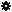﻿ Object Model: PolygonFaceCollection.NeighborPolygons

PolygonFaceCollection.NeighborPolygons

## PolygonFaceCollection.NeighborPolygonsv3.0

### Description

Returns a collection of PolygonFace objects for all neighbors within a given distance.

### C# Syntax

 ```PolygonFaceCollection PolygonFaceCollection.NeighborPolygons( Int32 in_lDistance ); ```

### Scripting Syntax

 ```oReturn = PolygonFaceCollection.NeighborPolygons( [Distance] ); ```

### Parameters

Parameter Type Description
Distance Integer Distance is an integer value representing the degree of neighborhood (eg: degree=2 for a polygon means its adjacent polygons plus the adjacent polygons of the adjacent polygons)

Default Value: 1

### Examples

#### VBScript Example

 ```set oCube = ActiveSceneRoot.AddGeometry( "Cube", "MeshSurface" ) set oGeometry = oCube.ActivePrimitive.Geometry set oVertices = oGeometry.Vertices set oNeighborPolygons = oVertices(0).NeighborPolygons set oNeighbors = oNeighborPolygons.NeighborPolygons str = "PolygonFace neighbors of this PolygonFaceCollection are :" for each polygon in oNeighbors str = str & " " & polygon.index next logmessage str ```